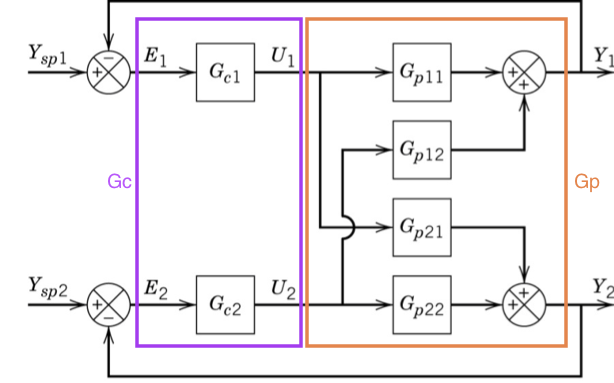# 82. Multivariable control¶

You may want to review the Transfer function matrix notebook before you look at this one.

## 82.1. Closed loop transfer functions for multivariable systems¶

Let’s consider a 2 $$\times$$ 2 system with feedback control as shown below::

import sympy
sympy.init_printing()

:

G_p11, G_p12, G_p21, G_p22, G_c1, G_c2 = sympy.symbols('G_p11, G_p12, G_p21, G_p22, G_c1, G_c2')


The matrix representation of the system is straigtforwardly handled by sympy.Matrix:

:

G_p = sympy.Matrix([[G_p11, G_p12],
[G_p21, G_p22]])


The controller is a bit harder. Convince yourself you understand how the off-diagonal elements of $$G_c$$ are zero in the diagram above.

:

G_c = sympy.Matrix([[G_c1, 0],
[0, G_c2]])


Now, we can redraw the block diagram using vectors for the signals and matrices for the blocks.Let’s derive the closed loop transfer function. There are three equations represented in the above diagram:

\begin{align} E &= Y_{sp} - Y\\ U &= G_c E = G_c(Y_{sp} - Y)\\ Y &= G_p U = G_p G_c(Y_{sp} - Y)\\ \end{align}

Now, we can solve for $$Y$$:

\begin{align} Y &= G_p G_c(Y_{sp} - Y)\\ Y &= G_p G_c Y_{sp} - G_p G_c Y \\ Y + G_p G_c Y&= G_p G_c Y_{sp} \\ (I + G_p G_c) Y &= G_p G_c Y_{sp} \\ Y &= (I + G_p G_c)^{-1} G_p G_c Y_{sp} \\ Y &= \Gamma Y_{sp} \end{align}

We can calculate the value of this function easily.

:

I = sympy.Matrix([[1, 0],
[0, 1]])

:

Gamma = sympy.simplify((I + G_p*G_c).inv()*G_p*G_c)

:

Gamma[0, 0]

:

$$\frac{G_{c1} \left(G_{c2} G_{p12} G_{p21} - G_{p11} \left(G_{c2} G_{p22} + 1\right)\right)}{G_{c1} G_{c2} G_{p12} G_{p21} - \left(G_{c1} G_{p11} + 1\right) \left(G_{c2} G_{p22} + 1\right)}$$
:

Gamma[0, 1]

:

$$- \frac{G_{c2} G_{p12}}{G_{c1} G_{c2} G_{p12} G_{p21} - \left(G_{c1} G_{p11} + 1\right) \left(G_{c2} G_{p22} + 1\right)}$$

We notice that there is a common divisor in all the elements of $$\Gamma$$. This is due to the calculation of the inverse of $$(I + G_p G_c)$$, which involves calculation of the determinant $$|I + G_p G_c|$$.

:

Delta = (I + G_p*G_c).det()
Delta

:

$$G_{c1} G_{c2} G_{p11} G_{p22} - G_{c1} G_{c2} G_{p12} G_{p21} + G_{c1} G_{p11} + G_{c2} G_{p22} + 1$$
:

(Delta*Gamma).simplify()

:

$$\left[\begin{matrix}G_{c1} \left(G_{c2} G_{p11} G_{p22} - G_{c2} G_{p12} G_{p21} + G_{p11}\right) & G_{c2} G_{p12}\\G_{c1} G_{p21} & G_{c2} \left(G_{c1} G_{p11} G_{p22} - G_{c1} G_{p12} G_{p21} + G_{p22}\right)\end{matrix}\right]$$

## 82.2. Characteristic equation¶

This leads us to conclude that we can calculate the characteristic equation of the closed loop transfer function as $$|I + G_p G_c|$$.

Notice that if we wanted the coupling the other way around, we could have worked with the same controller matrix permuted:

:

G_c*sympy.Matrix([[0, 1], [1, 0]])

:

$$\left[\begin{matrix}0 & G_{c1}\\G_{c2} & 0\end{matrix}\right]$$